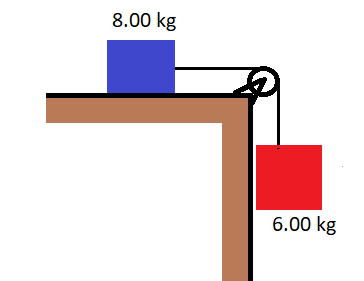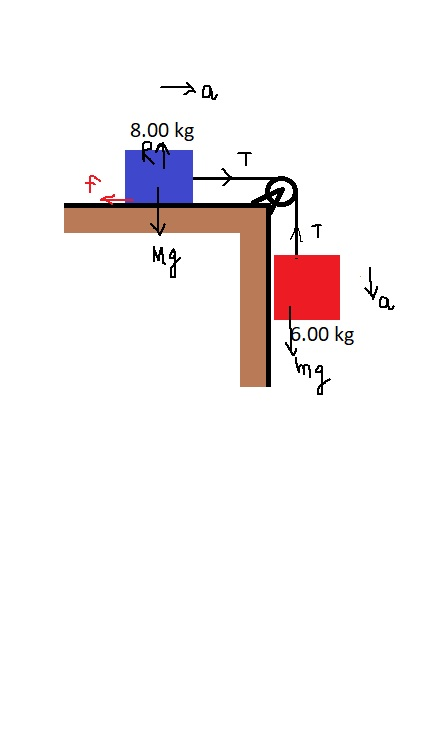# Consider the system shown in the figure. The rope and pulley have negligible mass, and the pulley...

## Question:

Consider the system shown in the figure. The rope and pulley have negligible mass, and the pulley is frictionless. The coefficient of kinetic friction between the 8.00-kg block and the tabletop is {eq}\mu_k {/eq}=0.250. Block of the 6.00 descends with constant velocity.

a) Draw free-body diagrams of both objects

b) Find the acceleration of the two objects

c) Find the tension in the string## Newton's Second law and Friction

According to Newton's second law, the net force on an object is given by the product of mass and acceleration of the object. If the object is at rest then force on it would be zero. And if the object is not in motion then static friction will come into act otherwise kinetic friction will be taking place.

Given

Mass of the block on the table (M) = 8 kg

Mass of the block hanging (m) = 6 kg

(a)

• R is the normal reaction
• T is the tension in the string
• f is friction force

We know that

{eq}\displaystyle f = \mu_{k}R {/eq}(b)

Considering the FBD of the mass m

{eq}\displaystyle mg - T = ma \\ T = mg - ma ----------(1) {/eq}

Now considering the FBD of mass M

{eq}\displaystyle T - f = Ma \\ T = f+ Ma ---------(2) {/eq}

Now from equation 1 and 2 , we can write

{eq}\displaystyle mg -ma = f + Ma \\ (6*9.81) - 6a = (\mu_{k}Mg) + Ma \\ 58.86 - 6a = (0.25*8*9.81) + 8a \\ 39.24 = 14 a \\ a = 2.8 \ m/s^{2} {/eq}

(c)

Now from the equation 1

{eq}\displaystyle T = mg -ma \\ T = 6(9.81 - 2.8) = 42.04 \ N {/eq}